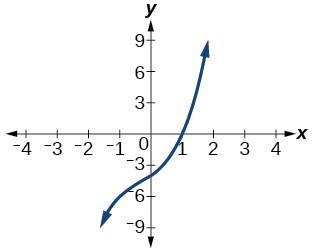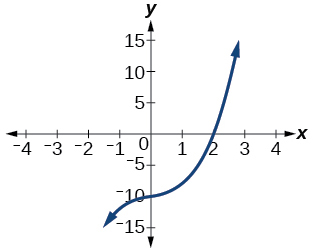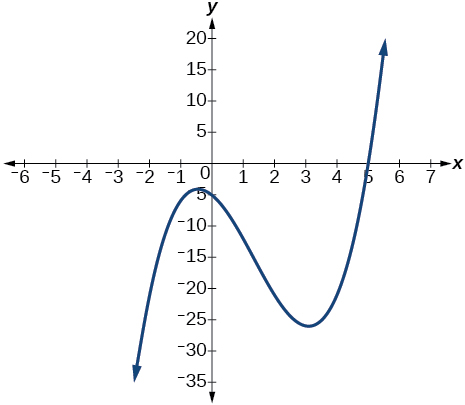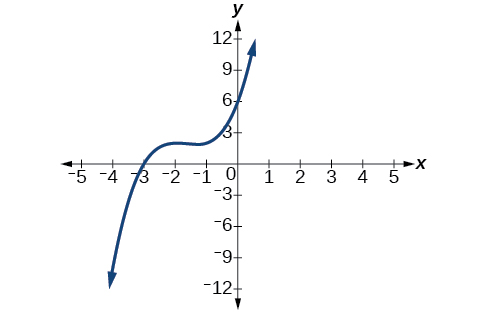# 5.4 Dividing polynomials  (Page 4/6)

 Page 4 / 6

## Verbal

If division of a polynomial by a binomial results in a remainder of zero, what can be conclude?

The binomial is a factor of the polynomial.

If a polynomial of degree $\text{\hspace{0.17em}}n\text{\hspace{0.17em}}$ is divided by a binomial of degree 1, what is the degree of the quotient?

## Algebraic

For the following exercises, use long division to divide. Specify the quotient and the remainder.

$\left({x}^{2}+5x-1\right)÷\left(x-1\right)$

$x+6+\frac{5}{x-1}\text{,}\text{\hspace{0.17em}}\text{quotient:}\text{\hspace{0.17em}}x+6\text{,}\text{\hspace{0.17em}}\text{remainder:}\text{\hspace{0.17em}}\text{5}$

$\left(2{x}^{2}-9x-5\right)÷\left(x-5\right)$

$\left(3{x}^{2}+23x+14\right)÷\left(x+7\right)$

$\left(4{x}^{2}-10x+6\right)÷\left(4x+2\right)$

$\left(6{x}^{2}-25x-25\right)÷\left(6x+5\right)$

$x-5\text{,}\text{\hspace{0.17em}}\text{quotient:}\text{\hspace{0.17em}}x-5\text{,}\text{\hspace{0.17em}}\text{remainder:}\text{\hspace{0.17em}}\text{0}$

$\left(-{x}^{2}-1\right)÷\left(x+1\right)$

$\left(2{x}^{2}-3x+2\right)÷\left(x+2\right)$

$2x-7+\frac{16}{x+2}\text{,}\text{\hspace{0.17em}}\text{quotient:}\text{​}\text{\hspace{0.17em}}2x-7\text{,}\text{\hspace{0.17em}}\text{remainder:}\text{\hspace{0.17em}}\text{16}$

$\left({x}^{3}-126\right)÷\left(x-5\right)$

$\left(3{x}^{2}-5x+4\right)÷\left(3x+1\right)$

$x-2+\frac{6}{3x+1}\text{,}\text{\hspace{0.17em}}\text{quotient:}\text{\hspace{0.17em}}x-2\text{,}\text{\hspace{0.17em}}\text{remainder:}\text{\hspace{0.17em}}\text{6}$

$\left({x}^{3}-3{x}^{2}+5x-6\right)÷\left(x-2\right)$

$\left(2{x}^{3}+3{x}^{2}-4x+15\right)÷\left(x+3\right)$

$2{x}^{2}-3x+5\text{,}\text{\hspace{0.17em}}\text{quotient:}\text{\hspace{0.17em}}2{x}^{2}-3x+5\text{,}\text{\hspace{0.17em}}\text{remainder:}\text{\hspace{0.17em}}\text{0}$

For the following exercises, use synthetic division to find the quotient.

$\left(3{x}^{3}-2{x}^{2}+x-4\right)÷\left(x+3\right)$

$\left(2{x}^{3}-6{x}^{2}-7x+6\right)÷\left(x-4\right)$

$2{x}^{2}+2x+1+\frac{10}{x-4}$

$\left(6{x}^{3}-10{x}^{2}-7x-15\right)÷\left(x+1\right)$

$\left(4{x}^{3}-12{x}^{2}-5x-1\right)÷\left(2x+1\right)$

$2{x}^{2}-7x+1-\frac{2}{2x+1}$

$\left(9{x}^{3}-9{x}^{2}+18x+5\right)÷\left(3x-1\right)$

$\left(3{x}^{3}-2{x}^{2}+x-4\right)÷\left(x+3\right)$

$3{x}^{2}-11x+34-\frac{106}{x+3}$

$\left(-6{x}^{3}+{x}^{2}-4\right)÷\left(2x-3\right)$

$\left(2{x}^{3}+7{x}^{2}-13x-3\right)÷\left(2x-3\right)$

${x}^{2}+5x+1$

$\left(3{x}^{3}-5{x}^{2}+2x+3\right)÷\left(x+2\right)$

$\left(4{x}^{3}-5{x}^{2}+13\right)÷\left(x+4\right)$

$4{x}^{2}-21x+84-\frac{323}{x+4}$

$\left({x}^{3}-3x+2\right)÷\left(x+2\right)$

$\left({x}^{3}-21{x}^{2}+147x-343\right)÷\left(x-7\right)$

${x}^{2}-14x+49$

$\left({x}^{3}-15{x}^{2}+75x-125\right)÷\left(x-5\right)$

$\left(9{x}^{3}-x+2\right)÷\left(3x-1\right)$

$3{x}^{2}+x+\frac{2}{3x-1}$

$\left(6{x}^{3}-{x}^{2}+5x+2\right)÷\left(3x+1\right)$

$\left({x}^{4}+{x}^{3}-3{x}^{2}-2x+1\right)÷\left(x+1\right)$

${x}^{3}-3x+1$

$\left({x}^{4}-3{x}^{2}+1\right)÷\left(x-1\right)$

$\left({x}^{4}+2{x}^{3}-3{x}^{2}+2x+6\right)÷\left(x+3\right)$

${x}^{3}-{x}^{2}+2$

$\left({x}^{4}-10{x}^{3}+37{x}^{2}-60x+36\right)÷\left(x-2\right)$

$\left({x}^{4}-8{x}^{3}+24{x}^{2}-32x+16\right)÷\left(x-2\right)$

${x}^{3}-6{x}^{2}+12x-8$

$\left({x}^{4}+5{x}^{3}-3{x}^{2}-13x+10\right)÷\left(x+5\right)$

$\left({x}^{4}-12{x}^{3}+54{x}^{2}-108x+81\right)÷\left(x-3\right)$

${x}^{3}-9{x}^{2}+27x-27$

$\left(4{x}^{4}-2{x}^{3}-4x+2\right)÷\left(2x-1\right)$

$\left(4{x}^{4}+2{x}^{3}-4{x}^{2}+2x+2\right)÷\left(2x+1\right)$

$2{x}^{3}-2x+2$

For the following exercises, use synthetic division to determine whether the first expression is a factor of the second. If it is, indicate the factorization.

$x-2,\text{\hspace{0.17em}}4{x}^{3}-3{x}^{2}-8x+4$

$x-2,\text{\hspace{0.17em}}3{x}^{4}-6{x}^{3}-5x+10$

Yes $\text{\hspace{0.17em}}\left(x-2\right)\left(3{x}^{3}-5\right)$

$x+3,\text{\hspace{0.17em}}-4{x}^{3}+5{x}^{2}+8$

$x-2,\text{\hspace{0.17em}}4{x}^{4}-15{x}^{2}-4$

Yes $\text{\hspace{0.17em}}\left(x-2\right)\left(4{x}^{3}+8{x}^{2}+x+2\right)$

$x-\frac{1}{2},\text{\hspace{0.17em}}2{x}^{4}-{x}^{3}+2x-1$

$x+\frac{1}{3},\text{\hspace{0.17em}}3{x}^{4}+{x}^{3}-3x+1$

No

## Graphical

For the following exercises, use the graph of the third-degree polynomial and one factor to write the factored form of the polynomial suggested by the graph. The leading coefficient is one.

Factor is $\text{\hspace{0.17em}}{x}^{2}-x+3$Factor is $\text{\hspace{0.17em}}\left({x}^{2}+2x+4\right)$$\left(x-1\right)\left({x}^{2}+2x+4\right)$

Factor is $\text{\hspace{0.17em}}{x}^{2}+2x+5$Factor is $\text{\hspace{0.17em}}{x}^{2}+x+1$$\left(x-5\right)\left({x}^{2}+x+1\right)$

Factor is ${x}^{2}+2x+2$For the following exercises, use synthetic division to find the quotient and remainder.

$\frac{4{x}^{3}-33}{x-2}$

$\text{Quotient:}\text{\hspace{0.17em}}4{x}^{2}+8x+16\text{,}\text{\hspace{0.17em}}\text{remainder:}\text{\hspace{0.17em}}-1$

$\frac{2{x}^{3}+25}{x+3}$

$\frac{3{x}^{3}+2x-5}{x-1}$

$\text{Quotient:}\text{\hspace{0.17em}}3{x}^{2}+3x+5\text{,}\text{\hspace{0.17em}}\text{remainder:}\text{\hspace{0.17em}}0$

$\frac{-4{x}^{3}-{x}^{2}-12}{x+4}$

$\frac{{x}^{4}-22}{x+2}$

$\text{Quotient:}\text{\hspace{0.17em}}{x}^{3}-2{x}^{2}+4x-8\text{,}\text{\hspace{0.17em}}\text{remainder:}\text{\hspace{0.17em}}-6$

## Technology

For the following exercises, use a calculator with CAS to answer the questions.

Consider $\text{\hspace{0.17em}}\frac{{x}^{k}-1}{x-1}\text{\hspace{0.17em}}$ with What do you expect the result to be if $\text{\hspace{0.17em}}k=4?$

Consider $\text{\hspace{0.17em}}\frac{{x}^{k}+1}{x+1}\text{\hspace{0.17em}}$ for What do you expect the result to be if $\text{\hspace{0.17em}}k=7?$

${x}^{6}-{x}^{5}+{x}^{4}-{x}^{3}+{x}^{2}-x+1$

Consider $\text{\hspace{0.17em}}\frac{{x}^{4}-{k}^{4}}{x-k}\text{\hspace{0.17em}}$ for What do you expect the result to be if $\text{\hspace{0.17em}}k=4?$

Consider $\text{\hspace{0.17em}}\frac{{x}^{k}}{x+1}\text{\hspace{0.17em}}$ with What do you expect the result to be if $\text{\hspace{0.17em}}k=4?$

${x}^{3}-{x}^{2}+x-1+\frac{1}{x+1}$

Consider $\text{\hspace{0.17em}}\frac{{x}^{k}}{x-1}\text{\hspace{0.17em}}$ with What do you expect the result to be if $\text{\hspace{0.17em}}k=4?$

## Extensions

For the following exercises, use synthetic division to determine the quotient involving a complex number.

$\frac{x+1}{x-i}$

$1+\frac{1+i}{x-i}$

$\frac{{x}^{2}+1}{x-i}$

$\frac{x+1}{x+i}$

$1+\frac{1-i}{x+i}$

$\frac{{x}^{2}+1}{x+i}$

$\frac{{x}^{3}+1}{x-i}$

${x}^{2}-ix-1+\frac{1-i}{x-i}$

## Real-world applications

For the following exercises, use the given length and area of a rectangle to express the width algebraically.

Length is $\text{\hspace{0.17em}}x+5,\text{\hspace{0.17em}}$ area is $\text{\hspace{0.17em}}2{x}^{2}+9x-5.$

Length is area is $\text{\hspace{0.17em}}4{x}^{3}+10{x}^{2}+6x+15$

$2{x}^{2}+3$

Length is $\text{\hspace{0.17em}}3x–4,\text{\hspace{0.17em}}$ area is $\text{\hspace{0.17em}}6{x}^{4}-8{x}^{3}+9{x}^{2}-9x-4$

For the following exercises, use the given volume of a box and its length and width to express the height of the box algebraically.

Volume is $\text{\hspace{0.17em}}12{x}^{3}+20{x}^{2}-21x-36,\text{\hspace{0.17em}}$ length is $\text{\hspace{0.17em}}2x+3,\text{\hspace{0.17em}}$ width is $\text{\hspace{0.17em}}3x-4.$

$2x+3$

Volume is $\text{\hspace{0.17em}}18{x}^{3}-21{x}^{2}-40x+48,\text{\hspace{0.17em}}$ length is $\text{\hspace{0.17em}}3x–4,\text{\hspace{0.17em}}$ width is $\text{\hspace{0.17em}}3x–4.$

Volume is $\text{\hspace{0.17em}}10{x}^{3}+27{x}^{2}+2x-24,\text{\hspace{0.17em}}$ length is $\text{\hspace{0.17em}}5x–4,\text{\hspace{0.17em}}$ width is $\text{\hspace{0.17em}}2x+3.$

$x+2$

Volume is $\text{\hspace{0.17em}}10{x}^{3}+30{x}^{2}-8x-24,\text{\hspace{0.17em}}$ length is $\text{\hspace{0.17em}}2,\text{\hspace{0.17em}}$ width is $\text{\hspace{0.17em}}x+3.$

For the following exercises, use the given volume and radius of a cylinder to express the height of the cylinder algebraically.

Volume is $\text{\hspace{0.17em}}\pi \left(25{x}^{3}-65{x}^{2}-29x-3\right),\text{\hspace{0.17em}}$ radius is $\text{\hspace{0.17em}}5x+1.$

$x-3$

Volume is $\text{\hspace{0.17em}}\pi \left(4{x}^{3}+12{x}^{2}-15x-50\right),\text{\hspace{0.17em}}$ radius is $\text{\hspace{0.17em}}2x+5.$

Volume is $\text{\hspace{0.17em}}\pi \left(3{x}^{4}+24{x}^{3}+46{x}^{2}-16x-32\right),\text{\hspace{0.17em}}$ radius is $\text{\hspace{0.17em}}x+4.$

$3{x}^{2}-2$

#### Questions & Answers

write down the polynomial function with root 1/3,2,-3 with solution
if A and B are subspaces of V prove that (A+B)/B=A/(A-B)
write down the value of each of the following in surd form a)cos(-65°) b)sin(-180°)c)tan(225°)d)tan(135°)
Prove that (sinA/1-cosA - 1-cosA/sinA) (cosA/1-sinA - 1-sinA/cosA) = 4
what is the answer to dividing negative index
In a triangle ABC prove that. (b+c)cosA+(c+a)cosB+(a+b)cisC=a+b+c.
give me the waec 2019 questions
the polar co-ordinate of the point (-1, -1)
prove the identites sin x ( 1+ tan x )+ cos x ( 1+ cot x )= sec x + cosec x
tanh`(x-iy) =A+iB, find A and B
B=Ai-itan(hx-hiy)
Rukmini
what is the addition of 101011 with 101010
If those numbers are binary, it's 1010101. If they are base 10, it's 202021.
Jack
extra power 4 minus 5 x cube + 7 x square minus 5 x + 1 equal to zero
the gradient function of a curve is 2x+4 and the curve passes through point (1,4) find the equation of the curve
1+cos²A/cos²A=2cosec²A-1
test for convergence the series 1+x/2+2!/9x3ByByByBy David MartinByByByBy Lakeima Roberts